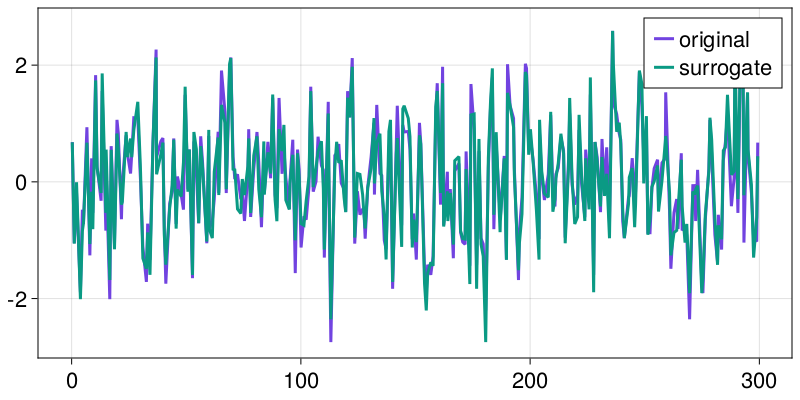# Surrogates for unevenly sampled time series

To derive a surrogate for unevenly sampled time series, we can use surrogate methods which which does not explicitly use the time axis like RandomShuffle or BlockShuffle, or we need to use algorithms that take the irregularity of the time axis into account.

## Lomb-Scargle based surrogate

The IrregularLombScargle surrogate is a form of a constrained surrogate which takes the Lomb-Scargle periodogram, which works on irregularly spaced data, to derive surrogates with similar phase distribution as the original time series. This function uses the simulated annealing algorithm[SchmitzSchreiber1999] to minimize the Minkowski distance between the original periodogram and the surrogate periodogram.

using TimeseriesSurrogates, CairoMakie, Random

# Example data: random AR1 process with a time axis with unevenly
# spaced time steps
rng = Random.MersenneTwister(1234)
x = AR1(n_steps = 300)
N = length(x)
t = (1:N) - rand(N)

# Use simulated annealing based on convergence of Lomb-Scargle periodograms
# The time series is relatively long, so set tolerance a bit higher than default.
ls = IrregularLombScargle(t, n_total = 100000, n_acc = 50000, tol = 5.0)
s = surrogate(x, ls, rng)

fig, ax = lines(t, x; label = "original")
lines!(ax, t, s; label = "surrogate")
axislegend(ax)
fig